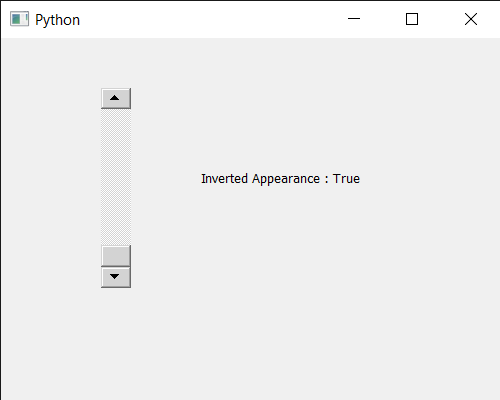# PyQt5 QScrollBar – Getting Inverted Appearance Property

• Last Updated : 30 Jul, 2020

In this article we will see how we can get inverted appearance property of QScrollBar. QScrollBar is a control that enables the user to access parts of a document that is larger than the widget used to display it. Slider is the scroll-able object inside the bar. This property holds whether or not a slider shows its values inverted. If this property is false (the default), the minimum and maximum will be shown in its classic position for the scroll bar. If the value is true, the minimum and maximum appear at their opposite location. It can be set with the help of `setInvertedAppearance` method.

In order to do this we will use `invertedAppearance` method with the scroll bar object.

Syntax : scroll.invertedAppearance()

Argument : It takes no argument

Return : It returns bool

Below is the implementation

 `# importing libraries``from` `PyQt5.QtWidgets ``import` `*` `from` `PyQt5 ``import` `QtCore, QtGui``from` `PyQt5.QtGui ``import` `*` `from` `PyQt5.QtCore ``import` `*` `import` `sys`` ` ` ` `class` `Window(QMainWindow):`` ` `    ``def` `__init__(``self``):``        ``super``().__init__()`` ` `        ``# setting title``        ``self``.setWindowTitle(``"Python "``)`` ` `        ``# setting geometry``        ``self``.setGeometry(``100``, ``100``, ``500``, ``400``)`` ` `        ``# calling method``        ``self``.UiComponents()`` ` `        ``# showing all the widgets``        ``self``.show()`` ` ` ` ` ` `    ``# method for components``    ``def` `UiComponents(``self``):`` ` `        ``scroll ``=` `QScrollBar(``self``)`` ` `        ``# setting geometry of the scroll bar``        ``scroll.setGeometry(``100``, ``50``, ``30``, ``200``)`` ` `        ``# making its background color to green``        ``scroll.setStyleSheet(``"background : lightgrey;"``)`` ` `        ``# setting inverted appearance``        ``scroll.setInvertedAppearance(``True``)`` ` `        ``# creating a label``        ``label ``=` `QLabel(``"GeesforGeeks"``, ``self``)`` ` `        ``# setting geometry to the label``        ``label.setGeometry(``200``, ``100``, ``300``, ``80``)`` ` `        ``# making label multi line``        ``label.setWordWrap(``True``)`` ` `        ``# getting value changed signal``        ``scroll.valueChanged.connect(``lambda``: do_action())`` ` `        ``# method called when signal is emitted``        ``def` `do_action():``             ` `            ``# setting text to the label``            ``label.setText(``"Current Value : "` `+` `str``(scroll.value()))`` ` `        ``# getting inverted appearance property``        ``value ``=` `scroll.invertedAppearance()`` ` `        ``# setting text to the label``        ``label.setText(``"Inverted Appearance : "` `+` `str``(value))`` ` ` ` ` ` ` ` ` ` `# create pyqt5 app``App ``=` `QApplication(sys.argv)`` ` `# create the instance of our Window``window ``=` `Window()`` ` `# start the app``sys.exit(App.``exec``())`

Output :My Personal Notes arrow_drop_up